American Journal of Networks and Communications
Volume 4, Issue 4, August 2015, Pages: 90-94

A Novel Hybrid Method for Face Recognition Based on 2d Wavelet and Singular Value Decomposition

Vahid Haji Hashemi1, *, Abdorreza Alavi Gharahbagh2

1Computer Engineering, Faculty of Engineering, Kharazmi University of Tehran,Tehran, Iran

2Department of Electrical and Computer Engineering, Islamic Azad University, Shahrood, Iran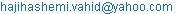(V. H. Hashemi)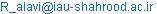(A. A. Gharahbagh)

Vahid Haji Hashemi, Abdorreza Alavi Gharahbagh. A Novel Hybrid Method for Face Recognition Based on 2d Wavelet and Singular Value Decomposition. American Journal of Networks and Communications. Vol. 4, No. 4, 2015, pp. 90-94. doi: 10.11648/j.ajnc.20150404.12

Abstract: An efficient face recognition system using eigen values of wavelet transform as feature vectors and radial basis function (RBF) neural network as classifier is presented. The face images are decomposed by 2-level two-dimensional (2-D) wavelet transformation. The wavelet coefficients obtained from the wavelet transformation are averaged for finding centers of features. In train process, four output of wavelet transform is analyzed and all eigenvalues of these images is obtained. At next step, the maximum 10 eigenvalues of wavelet sub images is stored as feature. Based on four sub images of wavelet transform and 10 eigenvalues of each sub image, the length of feature vector is 40. After obtaining features, in the train process for each person a center that has minimum Euclidean distance from all features is selected using RBF function. In fact the features are recognized by a RBF network. For a new input face image, firstly the feature vector is computed and then the distance (error) of this new vector with all centers of all persons is checked. The minimum distance is selected as target face. The proposed method on Essex face database and results showed that the proposed method provide better recognition rates with low computational complexity.

Keywords: Face Recognition, Singular Value Decomposition, SVD, Wavelet, Radial Basis Function, Neural Network

Contents

1. Introduction

Recognition of human faces is a very important task in many applications such as robotics, artificial intelligence, security systems etc. The wide category of face images such as its scene, brightness, lights, etc, are challenges for face detection algorithms. A face recognition system must be reliable and robust about all variable conditions of face images such as viewpoint, illumination, rotation, etc. The main tasks in the face recognition system are training with minimum face images and classification a new image based on its train process. Many researchers work about face recognition .In  face recognition methods classify to some groups. In the first group that named appearance based, total of face images or face objects are analyzed directly. Turk and Pent in  suggest using the eigenvalues in face recognition that is also popular than apparent base method. In  face matrix eigen values and vectors is computed and called Eigen faces, which are the principal components of face images. LDA1could be analyzed directly face images to extract the Fisher face  or analyzed the Eigen face to obtain a criteria for Eigen features of each face .Jain Yang et al. have developed a new technique by two-dimensional principal component analysis2 for image representation. 2DPCA is based on 2D image matrices so the image matrix does not need to be transformed into a vector . Rajagopalan proposeda system using multiple facial features extracted from the face .Image representation is a popular method in many image processing algorithms. Wavelet and FFT based feature extraction methods has many advantages. An appropriate wavelet transform is so robust regard to lighting changes and be capable of capturing substantial spatial features while has low computational complexity low. Bai-Ling Zhang use WaveletTransform and choosethe lowest resolution subband coefficients as face features . Another technique is WPD3that build a compact and meaningful feature and is also used in face recognition method . WPD is an extended form of the wavelet decomposition and includes multiple bases and different basis which result in better classification performance in compare to original wavelet .Ognian Boumbarov proposed a method that used wavelet packets for dimension reduction . Vytautas Perlibakas  has presented a work on face recognition using both PCA and WPD. In the face recognition process, classifier plays an important role. Conventional classifiers such as SVM, boosting method and neural networks have been employed in different classification methods.  proposed a hybrid approach that used NFP4 and NFS5 classifiers for robust decision in the presence of wide face image variations. The mostly network topology that used in face recognition methods as classifier is RBFNN6 due to its specifications and adaption to face features in comparison with other types of ANNs. HaiGuo and Jing-ying Zhao have proposed Chinese minority script recognition using radial basis function network . Many other researchers have implemented face recognition system using RBF neural network [15,16].Bicheng Li and Hujun Yin have presented a face recognition system using radial basis function neural network that its features is computed with wavelet transformation . Ning Jin and DerongLiu have developed WBFNN7with sequential learning algorithm and showed it has better performance in compare to sequential learning algorithm ofRBF neural network .In this paper, a face recognition methodbased on wavelet transform in combination with eigen value of 2d wavelet coefficients matrix for extracting features and radial basis function neural network as classifier is proposed. The wavelet packet transform is used as preprocessing step. The eigen values of matrixes of wavelet packet coefficients are computed. Based on SVD8 method the ten higher eigen values of each wavelet level is used as feature of image. The remainder of this paper is organized as follows. First, Section 2 describes preprocessing process. Section 3 presents the recognition process using RBFN. Section 4 describe results that showed improved correction accuracy andfinally, we conclude this paper and discuss our future work in Section 5.

2. Preprocessing and Feature Extraction

2.1. Preprocessing

Using the wavelet transformation, the image is mapped to a new time domain coordinates. This transform split the image in first level to four new sub images that the last matrix can mapped again in higher levels to four sub matrix. These new matrixes have many details about image and is so robust against noise and distortions. In the wavelet transformation, each new image has detailed coefficientsof the face that are decomposed as in Figure 1.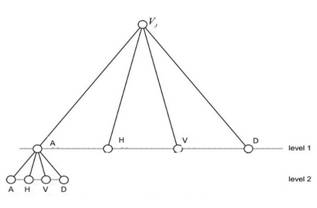Fig. 1. Wavelet transform structure.

The face image V is decomposed in level 1 to four new images. In these new sub images A is a resized image of original image that include all details, H is an approximated gradient of image in horizontal direction, V is approximated gradient of image in vertical direction and D is include diagonal details. If algorithm need more details, wavelet in level2 decompose A to four new sub images again and this routine can repeat until the size of resized image stored in A, is lower than a specified threshold. Likewise, horizontal, vertical and diagonal details are decomposed into the new sub images such as original images. During the wavelet transformation, at level 2, only the A is decomposed into approximation and details. In the proposed method the wavelet packet transformation has been applied on the face images for only two levels and obtained matrixes are including a resized original image with size about 25% of original face image, two horizontal details, two vertical details, and two diagonal details. The obtained wavelet packets in the preprocessing step is used for finding reliable features at next step as an input for recognition.

2.2. Feature Extraction

In the feature extraction step, firstly the eigen values and eigen vectors of all wavelet sub matrixes is extracted. At preprocessing step we extract seven sub matrixes from a face image. All eigen values and eigen vectors of these sub matrixes is computed. The eigenvectors of a matrix are the non-zero vectors which, after being multiplied by the matrix, remain proportional to the original vector, any vector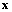that satisfies the equation: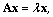where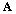is the wavelet sub image matrix,is the eigenvector and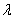is the associated eigenvalue. Eigenvectors are not unique for this reason usually pattern recognition methods use normalized eigen vectors that is a unique vector. If you consider a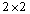matrix as a stretching, shearing or reflection transformation of the plane, you can see that the eigenvalues are the lines passing through the original matrix that are left unchanged by the transformation. In order to find the eigenvectors of a matrix we must start by finding the eigenvalues. The only way for finding eigen values is finding the zero value for determinant of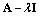. Once we have a set of eigenvalues we can substitute them back into the original equation to find the eigenvectors.

After finding all eigen values these values is sorted and ten maximum values is selected as the feature. In fact we summarize the total elements of a matrix in ten higher eigen values. In preprocessing step the wavelet transform implemented in level 2, split face image to seven submatrix. If we have select ten eigen value from each sub matrix we have a vector with length 7×10=70.

In train process, some face images of each person from different viewpoints, angles or scenes is selected and after preprocessing step the eigen values of wavelet sub matrixes from these images are extracted with above details as face features.

For eliminating noise or unwanted features from train process, after finding the features of a person face images, correlation coefficients between these vectors computed and if a vector is so different from other vectors or features is discard in train process. Another method that used for improving features performance is kmeans clustering method.

2.3. Kmeans for Noise Reduction

Kmeans clustering is a method of vector quantization, originally from signal processing that is popular for cluster analysis in image processing and data mining. Kmeansclustering aimsto map N observations or feature vector into k clusters or centers in which each observation belongs to the cluster with the nearest mean, distance or similarity.

This problem is computationally difficult however there are many efficient heuristic algorithms that are converge quickly to find centers. These method usually similar to the expectation-maximization algorithm for mixtures of Gaussian distributions however, kmeans clustering try to find clusters of comparable elements, while the expectation-maximization mechanism allows clusters to have different shapes. If the features is sparse or so different from each other and algorithm do not able to cluster all vectors in defined centers, the centers become diverge and algorithm missed centers. If a vector or features cause kmeans method to diverge, surely this feature is noise and should be discarded in train stage.

After analyzing all features accurately and eliminate weak and noisy features, in next step remaining features is used for training process.

3. RBFNN and Classification Step

The RBF network is a popular type of neural network including input, hidden and output layers with several forms of radial basis activation functions. Some of typical radial basis functions are Gaussian, Hardy Multiquadratic and Inverse Multiquadratic. The most common activation function is the Gaussian function defined by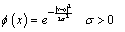Whereσ is the width parameter, μ is the vector determining the center of basis function and x is the ddimensional input vector. In a RBF network, a neuron of the hidden layer is activated whenever the input vector is close enough to its center vector μ in comparison the other centers. There are several techniques and heuristic methods for optimize and fast training the basis functions parameters and determining the number of hidden neurons needed to best classification. In the proposed algorithm two training algorithms is tested: FS9 and MM10.

In FS method one neuron is allocated to each group of features belong to each individual and if different faces of a person are not close to each other, more than one neuron will be necessary. The second training method MM is a mixture density model, whose parameters μ and σ are to be optimized by maximum likelihood ratio action. In this method the K basis functions is used as an input to the model that is typically is much less than the total number of input data points. In the output layer, RBF classifier comprises one neuron to each person. The final output are linear function of the outputs of the neurons in the hidden layer. The final classification decision is based on the greatest output. With RBF networks, the regions of the input space associated to each person can present an arbitrary form and disjoint regions can be associated to the same person to very different angles, viewpoints or different facial expressions.

As a mathematical expression of the output layer: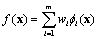Wherem is the number of hidden neurons, Wi are the weights connecting the hidden layer neurons and output layer neuron. The weights are adjusted using the following formulaWhere λ is a positive learning rate parameter and di is the desired output.

4. Simulation Results

The proposed method is tested on ORL face database. This standard database contains a set of face images taken between 1992 and 1994 at the Olivetti Research Lab in Cambridge, U.K, and include ten images for 40 individuals (totally 400 images). The images were taken with varying lighting, facial expressions (open and closed eyes, smiling or not smiling) and facial details (glasses or no glasses). All images has a dark homogeneous background with the person in an upright frontal position, with tolerance for some tilting and rotation of up to about 20 degrees. Scale varies about 10%. The original size of each image is 92x112 pixels, with 256 gray levels per pixel.

The proposed work has been carried out using Matlab 8.1. Figures 2 show the respective result of a face image after applying the wavelet transform in level 1.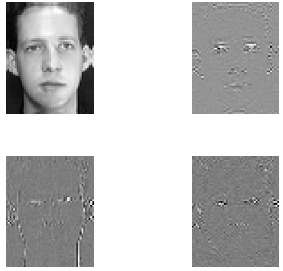Fig. 2. All wavelet results of a face image in level 1.

Different wavelet filters such as Haar, Symlet, Daubechies and Coifletswith different orders is tested in proposed method. The Eigen values of face imagesafter wavelet transforms is analyzed by correlation coefficients and kmeans method given in section 2. Features obtained using this processapplied as input to RBFN network. In the training process 70% of face images (7 images for each person) is used and remaining 30% is used as test. The recognition rates are obtained by doing the training and testing process repeatedly for 20 times and averaged to eliminate randomness of training process in RBFN. The results such as recognition rate, and average epoch number obtained from proposed method are shown in Table 1. As shown in table 1, the Daubechies type of wavelet with order 8 give the best features in our algorithm.

For RBFN neural network as classifier, the number of hidden layers is set to 10 and number of inputs equal to Eigen values vector size is 70.

5. Conclusion

A face recognition algorithm using the main eigen values of 2D wavelet matrixes, kmeans and correlation coefficient as preprocessing method and RBFN network as classifier is proposed. The simulation results using a database with 250 face images were carried out to compare the performance of the RBF classifiers and extracted features with other methods. The results indicated that the proposed method reach its maximumperformance around 96% of recognition rate. The RBF classifiers showed a better performance regarding toits sensitiveness to the choice of the training and testing sets.

Table 1. recognition rate as % and average epoch number of iterations in proposed method.

 Wavelet name Recognition Rate % Average Epoch number Haar 95.57 470.64 Sym4 90.16 474.45 Sym8 80.00 462.41 Sym12 82.63 464.41 Sym16 86.05 443.20 Db4 82.23 458.20 Db8 96.00 477.02 Db12 86.63 464.06 Db16 85.56 478.93 Coif2 81.72 448.14 Coif4 83.46 446.59

The important unknown parameters such as the number of hidden layers in RBFN, number of eigen values selected from each wavelet matrix, level of wavelet transform and its filter type is set based on experimental results. Future work can be evaluate the robustness of ROI based methods in face recognition such as methods extract eyes, nose, lip and ears and analyze separately these elements.

References

1. W. Zhao, R. Chellappa, A. Rosenfeld and P. J. Phillips, "Face Recognition: A Literature Survey," Technical Re- port CAR-TR-948, University of Maryland, College Park, 2000.
2. M. Turk and A. Pentland, "Eigen faces for Recognition," Cognitive Neuroscience, Vol. 3, No. 1, 1991, pp. 71-86.
3. P. Belhumeur, J. Hespanha and D. Kriegman, "Eigen faces vs Fisher Faces: Recognition Using Class Specific Linear Projection," IEEE Transactions on Pattern Analysis and Machine Intelligence, Vol. 20, No. 7, 1997, pp. 711-720.
4. D. L. Swets and J. Weng, "Using Discriminant Eigen features for Image Retrieval," IEEE Transactions on Pattern Analysis and Machine Intelligence, Vol. 18, No. 8, 1996, pp. 831-836.
5. J. Yang, D. Zhang, A. F. Frangi and J.-Y. Yang, "Two- Dimensional PCA: A New Approach to Appearance- Based Face Representation and Recognition," IEEE Transactions on Pattern Analysis and Machine Intelligence, Vol. 26, No. 1, 2004, pp. 131-138.
6. A. N. Rajagopalan, K. S. Rao and Y. A. Kumar, "Face Recognition Using Multiple Facial Features," Pattern Recognition Letters, Vol. 28, No. 3, 2007, pp. 335-341.
7. B.-L. Zhang, H. H. Zhang and S. Z. S. Ge, "Face Recognition by Applying Wavelet Sub band Representation and Kernel Associative Memory," IEEE Transactions on Neural Networks, Vol. 15, No. 1, 2005, pp. 166-177
8. C. Garcia, G. Zikos and G. Tziritas, "Wavelet Packet Analysis for Face Recognition," Image and Vision Computing, Vol. 18, No. 4, 2000, pp. 289-297.
9. J. Z. Xue, H. Zhang and C. X. Zheng, "Wavelet Packet Transform for Feature Extraction of EEG during Mental Tasks," Proceedings of the Second International Confer- ence on Machine Learning and Cybernetics, Vol. 1, 2003, pp. 360-363.
10. O. Boumbarov, S. Sokolov and G. Gluhchev, "Combined Face Recognition Using Wavelet Packets and Radial Ba- sis Function Neural Network," International Conference on Computer Systems and Technologies—CompSysTech’07, Bulgaria, 14-15 June 2007, pp. v.4.1-v.4.7.
11. V. Perlibakas, "Face Recognition Using Principal Component Analysis and Wavelet Packet Decomposition," Informatica, Vol. 15, No. 2, 2004, pp. 243-250.
12. J.-T. Chien and C.-C. Wu, "Discriminant Wavelet faces and Nearest Feature Classifiers for Face Recognition," IEEE Transactions on Pattern analysis and Machine Intelligence, Vol. 24, No. 12, 2002, pp. 1644-1649.
13. T. M. Mitchell, "Machine Learning," China Machine Press, Beijing, 2003.
14. H. Guo and J.-Y. Zhao, "Chinese Minority Script Recognition Using Radial Basis Function Network," Journal of Computers, Vol. 5, No. 6, 2010, pp. 927-934.
15. X.-Y. Jing, Y.-F. Yao, J.-Y. Yang and D. Zhang, "A Novel Face Recognition Approach Based on Kernel Dis- criminative Common Vectors (KDCV) Feature Extraction and RBF Neural Network," Neuro computing, Vol. 71, No. 13-15, 2008, pp. 3044-3048.
16. M. J. Er, S. Q. Wu, J. W. Lu and H. L. Toh, "Face Recognition with Radial Basis Function (RBF) Neural Net- works," IEEE Transactions on Neural Networks, Vol. 13, No. 3, 2002, pp. 697-710.
17. B. C. Li and H. J. Yin, "Face Recognition Using RBF Neural Networks and Wavelet Transform," Lecture Notes in Computer Science, Vol. 3497, 2005, pp. 105-111.
18. N. Jin and D. R. Liu, "Wavelet Basis Function Neural Networks for Sequential Learning," IEEE Transactions on Neural Networks, Vol. 19, No. 3, 2008, pp. 523-528.

Footnotes

 Linear Discriminant Analysis

 2DPCA

 Wavelet Packet Decomposition

nearestfeature plane

 nearest feature space

 radial basis function neural network

 wavelet basis function neural network

 Singular value decomposition

Forward selection

Gaussian mixture model

 Contents 1. 2. 2.1. 2.2. 2.3. 3. 4. 5.
Article ToolsAbstractPDF(230K)